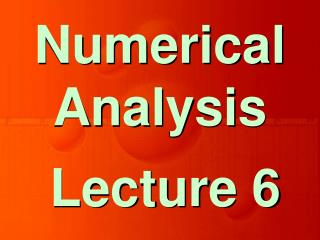# Lecture 6 - PowerPoint PPT PresentationDownload PresentationLecture 6

Lecture 6Download Presentation## Lecture 6

- - - - - - - - - - - - - - - - - - - - - - - - - - - E N D - - - - - - - - - - - - - - - - - - - - - - - - - - -
##### Presentation Transcript

1. Numerical Analysis Lecture 6

2. Chapter 2 Solution of Non-Linear Equations

3. IntroductionBisection MethodRegula-Falsi MethodMethod of iterationNewton - Raphson MethodSecant MethodMuller’s MethodGraeffe’s Root Squaring Method

4. Bisection Method (Bolzano)

5. Regula-Falsi Method Method of false position

6. Method of Iteration

7. Let x = a is the desired root Suppose x0 is its initial approximation. The first and successive approximations to the root can be obtained as

8. Newton -Raphson Method

9. An approximation to the root is given by

10. Better and successive approximations x2, x3, …, xn to the root are obtained from N-R Formula

11. Example Find a real root of the equation x3 – x – 1 = 0 using Newton - Raphson method, correct to four decimal places.

12. Solution Let f (x) = x3 –x – 1, Then f (1) = – 1, f (2) = 5 Therefore, the root lies in the interval (1, 2).

13. Further f’ (x) = 3x2 – 1, f” (x) =6x Since f (2) and f”(2) are of the same sign, we choose x0 = 2 as the first approximation to the root.

14. The second approximation is computed using Newton-Raphson method as • and

15. The successive approximations are • Hence, the required • root is 1.3247.

16. Note ! • Methods such as bisection method and the false position method of finding roots of a nonlinear equation f( x ) = 0 require bracketing of the root by two guesses. Such methods are called bracketing methods.

17. These methods are always convergent since they are based on reducing the interval between the two guesses to zero in on the root.

18. In the Newton-Raphson method, the root is not bracketed. Only one initial guess of the root is needed to get the iterative process started to find the root of an equation. Hence, the method falls in the category of open methods.

19. Newton - Raphson method is based on the principle that if the initial guess of the root of f( x ) = 0 is at xi, then if one draws the tangent to the curve at f( xi ), the point xi+1 where the tangent crosses the x-axis is an improved estimate of the root

20. Draw backs of N-R Method Divergence at inflection points: If the selection of a guess or an iterated value turns out to be close to the inflection point of f ( x ), [where f”( x ) = 0 ], the roots start to diverge away from the root.

21. Division of zero or near zero: • If an iteration, we come across the division by zero or a near-zero number, then we get a large magnitude for the next value, xi+1.

22. Root jumping: In some case where the function f (x) is oscillating and has a number of roots, one may choose an initial guess close to a root. However, the guesses may jump and converge to some other root.

23. Oscillations near local maximum and minimum: Results obtained from N-R method may oscillate about the local max or min without converging on a root but converging on the local max or min. Eventually, it may lead to division to a number close to zero and may diverge.

24. Convergence of N-R Method

25. Let us compare the N-R formula • with the general iteration formula

26. The iteration method converges if • Therefore, N-R formula converges, provided • in the interval considered.

27. Newton-Raphson formula therefore converges, provided the initial approximation x0 is chosen sufficiently close to the root and are continuous and bounded in any small interval containing the root.

28. Definition • Let • where is a root of f (x) = 0. • If we can prove that • where K is a constant and is the error involved at the n - th step, while finding the root by an iterative method, then the rate of convergence of the method is p.

29. The N-R method converges quadratic ally where is a root of f (x) = 0 and is the error involved at the n-th step, while finding the root by N-R formula

30. Using Taylor’s expansion, we get

31. Since is a root, Therefore, the above expression simplifies to

32. On neglecting terms of order and higher powers, we obtain • Where • It shows that N-R method has second order convergence or converges quadratic ally.

33. Example • Set up Newton’s scheme of iteration for finding the square root of a positive number N.

34. Solution • The square root of N can be carried out as a root of the equation • Let

35. By Newton’s method, we have • In this Problem • Therefore

36. Example • Evaluate , by Newton’s formula. • Solution • Since • we take

37. Hence

38. Numerical Analysis Lecture 6